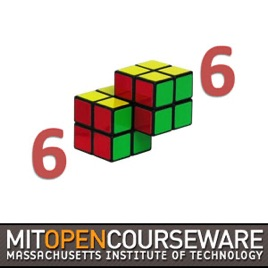47 episodes

Lecture videos from 6.006 Introduction to Algorithms, taught by Erik Demaine and Srini Devadas. The course is divided into eight units: introduction, sorting and trees, hashing, numerics, graphs, shortest paths, dynamic programming, and advanced topics.# Introduction to Algorithms (2011) MIT

• Technology

Lecture videos from 6.006 Introduction to Algorithms, taught by Erik Demaine and Srini Devadas. The course is divided into eight units: introduction, sorting and trees, hashing, numerics, graphs, shortest paths, dynamic programming, and advanced topics.

5.0 out of 5
1 Rating

1 Rating